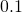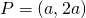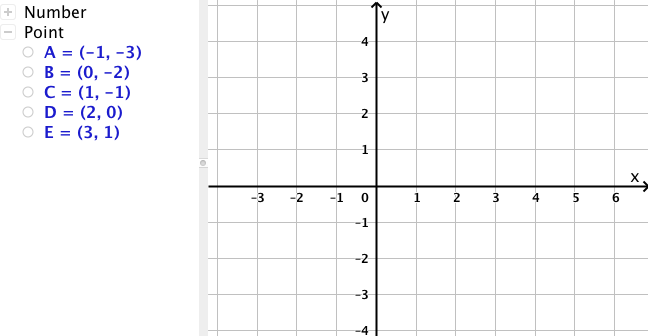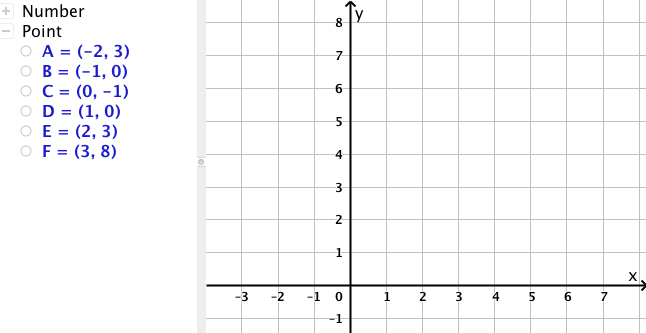# Graphing Relations on the Cartesian Plane

When both sets of a relation, the domain and the range, are numerical, it is often helpful to graph the relation. The idea of graphing on a two dimensional plane (the Cartesian Plane) came from René Descartes, who lived 1596 – 1650.

# Generating Many Points: using a slider

For printing: Graphing Relations on the Cartesian Plane

On this applet, a set of points is drawn. A slider is also drawn showing values fromtogoing up in steps of. Right now, when you click and drag the slider, not much happens.

Notice that thecoordinate is twice thecoordinate. Each point follows the pattern.

We can create a dynamic point using the slider that will travel through all of our points, because of this simple arithmetic relationship between theandcoordinates.

In the input bar, enter a point as follows:Make sure to use uppercase P. Any other uppercase letter will work, just avoid using letters already used. Now drag the handle on the slider to see what happens. Your new point P should go through given points.

You can right click on the point P to ‘show trace’.For each set of points below (or on the worksheet), first move the points on the applet to the correct location,. Then figure out the simple arithmetic relationship between thecoordinate and thecoordinate. Finally, edit the point P so that it will go through the set of points.

Set 1:Set 2:Set 3:Set 4:Set 5:Set 6:Next page: Domain and Range